Courses

# Notes | EduRev

## NEET : Notes | EduRev

The document Notes | EduRev is a part of the NEET Course Physics 28 Years Past year papers for NEET/AIPMT Class 11.
All you need of NEET at this link: NEET

Q 1. The speed of a swimmer in still water is 20 m/s. The speed of river water is 10 m/s and is flowing due east. If he is standing on the south bank and wishes to cross the river along the shortest path, the angle at which he should make his strokes w.r.t. north is given by:    
A: 30° west
B: 0°
C: 60° west
D: 45° west
Ans:
A
Solution:
VSR = 20m/s
VRG =  10m/s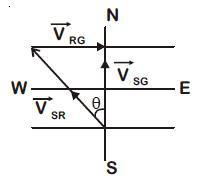sin θ = 10/20
sin θ = 1/2
θ = 300 west

Q 2. A particle moving with velocity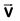is acted by three forces shown by the vector triangle PQR. The velocity of the particle will: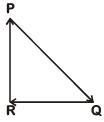A: Increase
B: Decrease
C: Remain constant
D: Change according to the smallest force
Ans:
C
Solution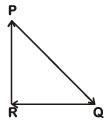As forces are forming closed loop in same order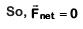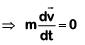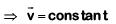Q 3. The x and y coordinates of the particle at any time are x = 5t – 2t2 and y = 10t respectively, where x and y are in meters and t in seconds. The acceleration of the particle at t = 2s is:-    
A: 5 m/s2
B: – 4 m/s2
C: – 8 m/s2
D: 0
Ans:
B
Solution: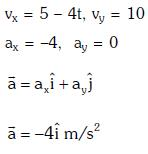Q 4. One end of string of length l is connected to a particle of mass 'm' and the other end is connected to a small peg on a smooth horizontal table. If the particle moves in circle with speed 'v' the net force on the particle (directed towards centre) will be (T represents the tension in the string):-    
A: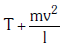B: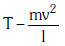C: Zero
D: T
Ans:
D
Solution: Net force on the particle in uniform circular motion is centripetal force, which is provided by the tension in string.

Q 5. If the magnitude of sum of two vectors is equal to the magnitude of difference of the two vectors, the angle between these vectors is:    
A: 180 deg
B: 0 deg
C: 90 deg
D: 45 deg
Ans:
C
Solution: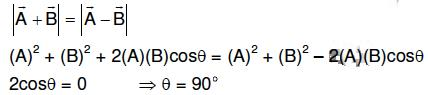Q 6. A ship A is moving Westwards with a speed of 10 km h-1 and a ship B 100 km South of A, is moving Northwards with a speed of 10 km h-1. The time after which the distance between them becomes shortest, is:    
A: 10√2h
B: 0 h
C: 5h
D: 5√2h
Ans:
C
Solution: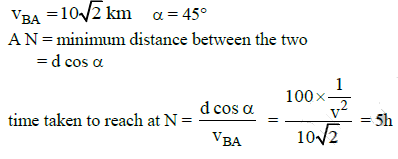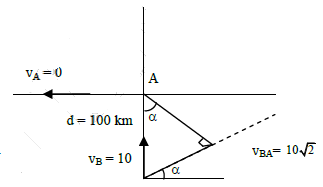Q 7. A particle is moving such that its position coordinates (x, y) are (2m, 3m) at time t = 0, (6m, 7m) at time t = 2s and (13m, 14m) at time t= 5s. Average velocity vector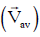from t = 0 to t = 5s is:    
A: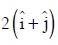B: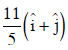C: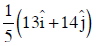D: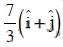Ans:
B
Solution:
Average velocity Vector,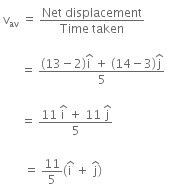Offer running on EduRev: Apply code STAYHOME200 to get INR 200 off on our premium plan EduRev Infinity!

,

,

,

,

,

,

,

,

,

,

,

,

,

,

,

,

,

,

,

,

,

;